GeometryShowing results 21 to 30 of 51, on page 3 of 6 Area Coordinate Systems Curves and Shapes Length/Perimeter Volume
 DESCRIPTION EQUATION Equation of a Circle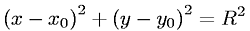Equation of a Hyperbola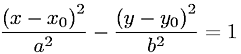Equation of a Hyperbolic Paraboloid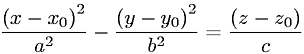Equation of a Hyperboloid of One Sheet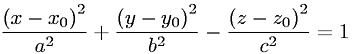Equation of a Hyperboloid of Two Sheets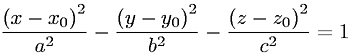Equation of a Line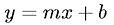Equation of a ParabolaEquation of a PlaneEquation of a SphereEquation of an Ellipse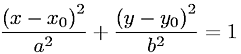Go to page: 1  2  3  4  5  6